# Voltage Regulator Circuits

Published  July 27, 2018   0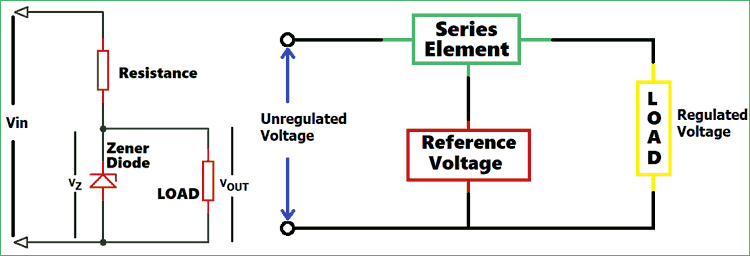Voltage Regulator, as name suggests, is a circuit which is used to regulate the voltage. Regulated voltage is smooth supply of voltage, free from any noise or disturbance. The output from voltage regulator is independent of load current, temperature and AC line variation. Voltage regulators are present in almost every electronics or house hold appliances like TV, Fridge, computer etc, to stabilize the supply voltage.

Basically, voltage regulator minimizes the variation in voltage to protect the device. In electrical distribution system, the voltage regulators are either in feeder lines or at substation. There are two types of regulators used in this line, one is step regulator, in which switches regulate the current supply. Another one is induction regulator, which is an alternating electrical machine similar to an induction motor supplies power as a secondary source. It minimizes the voltage variation and provide stable output.

There are different types of voltage regulators which are explained below.

### Types of Voltage Regulator Circuit

Linear Voltage Regulator Circuit

• Series Voltage Regulator
• Shunt Voltage Regulator

Zener Voltage Regulator Circuit

Switching Voltage Regulator Circuit

• Buck type
• Boost type
• Buck/Boost type

### Linear Voltage Regulator Circuit

These are the most common regulators used in electronics to maintain the steady output voltage. Linear voltage regulators acts like a voltage divider circuit, in this regulator resistance varies with respect to change in load and gives constant output voltage. Some advantages and disadvantages of linear voltage regulator are given below:

• Output ripple voltage is low
• Response is fast
• Less noise

• Low efficiency
• Large space required
• Output voltage will always be less than input voltage

### 1. Series Voltage Regulator

Series Voltage Regulator is a part of Linear Voltage Regulator and also called as Series Pass Regulator. A variable element connected in series, used for maintaining constant output voltage. As you change the resistance of series element voltage drop across it can be varied to ensure that the voltage across output is constant.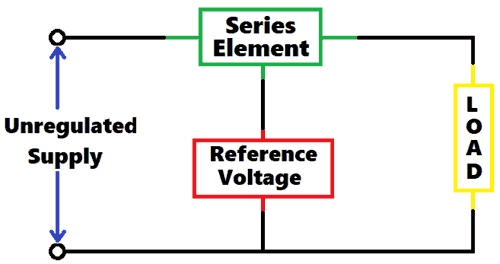As you can see the circuit diagram for Series Voltage Regulator, NPN transistor T1 is the series element and a zener diode is used to provide the reference voltage.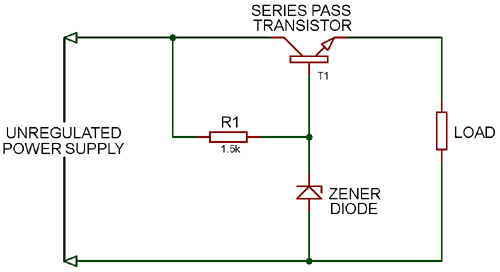When the output voltage increases the base-emitter voltage decreases, due to this transistor T1 conduct less. As T1 conducts less it reduce the output voltage hence maintains the output voltage constant.

When the output voltage decreases the base-emitter voltage increases, due to this transistor T1 conduct more. As T1 conducts more it increase the output voltage hence maintains the output voltage constant.

The output voltage is defined as:

```VO = VZ - VBE
Where,
VO is the output voltage
VZ is Zener breakdown voltage
VBE is base-emitter voltage```

### 2. Shunt Voltage Regulator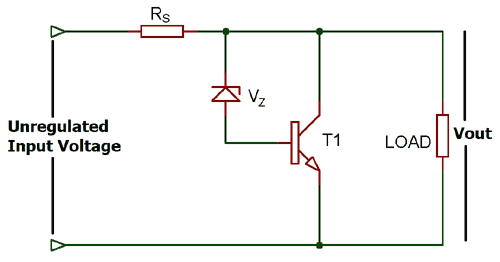The unregulated voltage is directly proportional to the voltage drop across the resistance connected in series and this voltage drop depends upon the current consumed by the load. If the current consumption of load increases the base current will also decrease and due to this less collector current will flow through the collector emitter terminal and hence the current through load will increase and vice versa.

The regulated output voltage of shunt voltage regulator is defined as:

`VOUT = VZ + VBE`

### Zener Voltage Regulator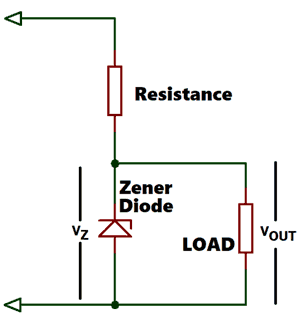Zener Voltage Regulators are cheaper and only suitable for low power circuits. It can be used in applications where the amount of power wasted during regulation is not of major concern.

A resistor, is connected in series with the zener diode to limit the amount of current flowing through the diode and the input voltage Vin (Which must be greater than the zener voltage) is connected across as shown in the image and the output voltage Vout, is taken across the zener diode with Vout = Vz (Zener Voltage). As we know Zener diode starts conducting in reverse direction when the applied voltage is higher than the breakdown voltage of Zener. So when its start conducting, it maintains the same voltage across it and flow back the extra current, thus provide the stable output voltage.

### Switching Voltage Regulator

There are three types of switching voltage regulator:

• Buck or Step-Down Switching Voltage Regulator
• Boost or Step-Up Switching Voltage Regulator
• Buck / Boost Switching Voltage Regulator

### Buck or Step-Down Switching Voltage Regulator

A Buck Regulator is used to step down the voltage at the output, we can even use the voltage divider circuit to reduce the output voltage but the efficiency of voltage divider circuit is low, because resistors dissipates energy as heat. We use capacitor, diode, inductor and switch in the circuit. The circuit diagram for Buck Switching Voltage Regulator is given below: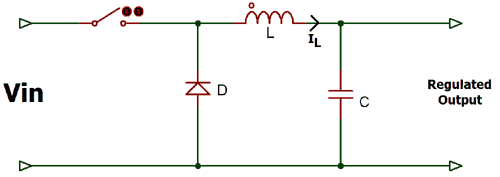When the switch in ON the diode remain reversed biased and power supply is connected to the inductor. When the switch is open the polarity of inductor get reverse and the diode become forward biased and connect the inductor to the ground. Then the current through the inductor decreases with slope:

`d IL / dt = (0-VOUT) / L`

The Capacitor is used to prevent the voltage from dropping to zero across the load. If we keep opening and closing switch the average voltage across the load will be less than the supplied input voltage. You can control the output voltage by varying the duty cycle of the switching device.

`Output Voltage = (Input Voltage) * (percentage of time that the switch is ON)`

### Boost or Step-Up Switching Voltage Regulator

The Boost Regulator is used to step-up the voltage across the load. The circuit diagram for boost regulator is given below: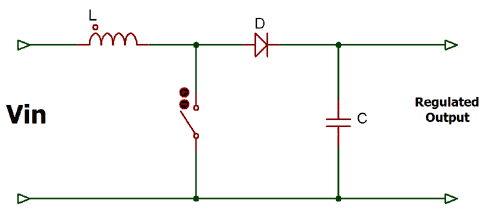When the switch is closed the diode behaves as reversed biased and the current across the inductor keeps increasing. Now when the switch is opened, the inductor will create a force causing the current to continue flowing and capacitor starts charging. By continuous turning the switch ON and OFF we will receive the voltage at the load higher than the input voltage. We can control the output voltage by controlling the turn ON (Ton) time of the switch.

`Output Voltage = Input Voltage / Percentage of time that the switch is open`

### Buck-Boost Switching Voltage Regulator

Buck-Boost Switching Regulator is the combination of both Buck and Boost Regulator, it gives inverted output which can be greater or less than the supplied input voltage.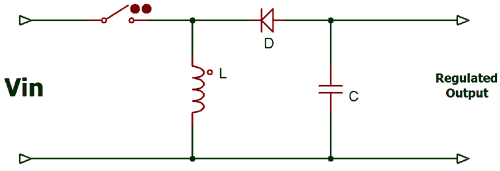When the switch is ON the diode behaves as reversed biased and inductor stores energy and when the switch is OFF inductor start releasing the energy with the reverse polarity, which charges the capacitor. When the energy stored in inductor becomes zero the capacitor starts discharging into the load with reverse polarity. Due to this buck-boost regulator also called as inverting regulator.

The output voltage is defined as

```Vout = Vin (D / 1-D)
Where, D is the Duty cycle```

Hence, if the Duty Cycle is low the regulator behaves as the Buck Regulator and when the Duty Cycle is high the regulator behaves as the Boost Regulator.

### Practical Example for Regulator Circuits

Positive Linear Voltage Regulator Circuit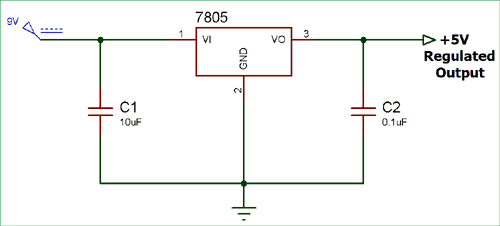We have designed a positive linear voltage regulator circuit using 7805 IC. This IC has all the circuitry to provide the 5volt regulated supply. The input voltage should be atleast more than 2v from the rated value like for LM7805 we should atleast provide 7v.

Unregulated input voltage is supplied to the IC and we get regulated voltage at the output terminal. The name of the IC defines its function, 78 represent the positive sign and 05 represents the value of the regulated output voltage. As you see in the circuit diagram we are giving 9V to the 7805IC and getting regulated +5V at the output. The capacitor C1 and C2 are used for filtration.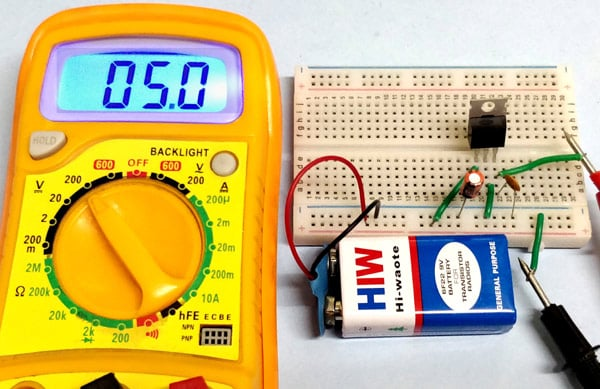Zener Voltage Regulator Circuit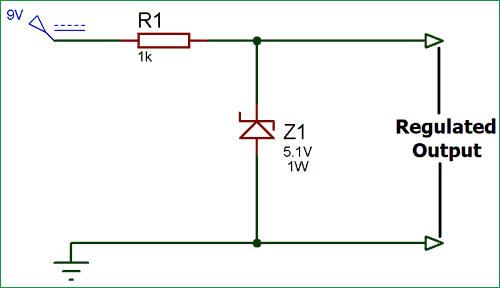Here, we have designed a Zener Voltage Regulator using 5.1V of Zener diode. The Zener diode works as the sensing element. When the supply voltage exceeds its breakdown voltage, its start conducting in reverse direction and maintains the same voltage across it and flow back the extra current, thus provide the stable output voltage. In this circuit we are giving 9V of input voltage and getting nearly 5.1 voltage of regulated output.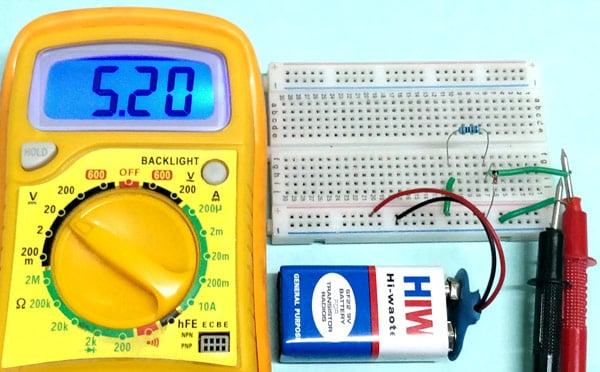Video